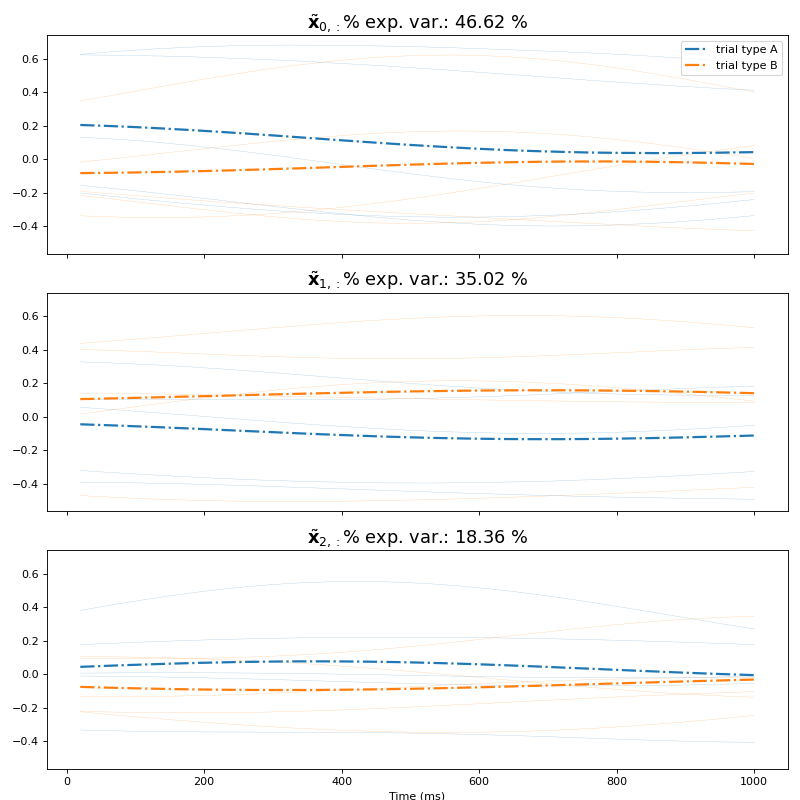# viziphant.gpfa.plot_dimensions_vs_time¶

viziphant.gpfa.plot_dimensions_vs_time(returned_data, gpfa_instance, dimensions='all', orthonormalized_dimensions=True, n_trials_to_plot=20, trial_grouping_dict=None, colors='grey', plot_single_trajectories=True, plot_group_averages=False, n_columns=2, plot_args_single={'alpha': 0.4, 'linestyle': '-', 'linewidth': 0.3}, plot_args_average={'alpha': 1, 'linestyle': 'dashdot', 'linewidth': 2}, figure_args={'figsize': (10, 10)})[source]

This function plots all latent space state dimensions versus time. It is a wrapper for the function plot_single_dimension_vs_time and places the single plot onto a grid.

Optional visual aids are offered such as grouping the trials and color coding their traces. Changes to optics of the plot can be applied by providing respective dictionaries.

This function is an adaption of the MATLAB implementation by Byron Yu which was published with his paper: Yu et al., J Neurophysiol, 2009.

Parameters
returned_datanp.ndarray or dict

When the length of returned_data is one, a single np.ndarray, containing the requested data (the first entry in returned_data keys list), is returned. Otherwise, a dict of multiple np.ndarrays with the keys identical to the data names in returned_data is returned.

N-th entry of each np.ndarray is a np.ndarray of the following shape, specific to each data type, containing the corresponding data for the n-th trial:

latent_variable_orth: (#latent_vars, #bins) np.ndarray

latent_variable: (#latent_vars, #bins) np.ndarray

y: (#units, #bins) np.ndarray

Vsm: (#latent_vars, #latent_vars, #bins) np.ndarray

VsmGP: (#bins, #bins, #latent_vars) np.ndarray

Note that the num. of bins (#bins) can vary across trials, reflecting the trial durations in the given spiketrains data.

gpfa_instanceGPFA

Instance of the GPFA() class in elephant, which was used to obtain returned_data.

dimensions‘all’ or int or list of int, optional

Dimensions to plot. Default: ‘all’

orthonormalized_dimensionsbool, optional

Boolean which specifies whether to plot the orthonormalized latent state space dimension corresponding to the entry ‘latent_variable_orth’ in returned data (True) or the unconstrained dimension corresponding to the entry ‘latent_variable’ (False). Beware that the unconstrained state space dimensions ‘latent_variable’ are not ordered by their explained variance. These dimensions each represent one Gaussian process timescale \$ au\$. On the contrary, the orthonormalized dimensions ‘latent_variable_orth’ are ordered by decreasing explained variance, allowing a similar intuitive interpretation to the dimensions obtained in a PCA. Due to the orthonormalization, these dimensions reflect mixtures of timescales. Default: True

n_trials_to_plotint, optional

Number of single trial trajectories to plot. Default: 20

trial_grouping_dictdict or None

Dictionary which specifies the groups of trials which belong together (e.g. due to same trial type). Each item specifies one group: its key defines the group name (which appears in the legend) and the corresponding value is a list or np.ndarray of trial IDs. Default: None

colorsstr or list of str, optional

List of strings specifying the colors of the different trial groups. The length of this list should correspond to the number of items in trial_grouping_dict. In case a string is given, all trials will share the same color unless trial_grouping_dict is specified, in which case colors will be set automatically to correspond to individual groups. Default: ‘grey’

plot_single_trajectoriesbool, optional

If True, single trial trajectories are plotted. Default: True

plot_group_averagesbool, optional

If True, trajectories of those trials belonging together specified in the trial_grouping_dict are averaged and plotted. Default: False

n_columnsint, optional

Number of columns of the grid onto which the single plots are placed. The number of rows are deduced from the number of dimensions to be plotted. Default: 2

plot_args_singledict, optional

Arguments dictionary passed to ax.plot() of the single trajectories.

plot_args_averagedict, optional

Arguments dictionary passed to ax.plot() of the average trajectories.

figure_argsdict, optional

Arguments dictionary passed to matplotlib.pyplot.figure(), if ax is None.

Returns
figmatplotlib.figure.Figure
axesmatplotlib.axes.Axes

Examples

In the following example, we calculate the neural trajectories of 20 independent Poisson spike trains recorded in 50 trials with randomized rates up to 100 Hz and plot the resulting orthonormalized latent state space dimensions.

```import numpy as np
import quantities as pq
from elephant.gpfa import GPFA
from elephant.spike_train_generation import homogeneous_poisson_process
from viziphant.gpfa import plot_dimensions_vs_time
np.random.seed(24)
n_trials = 10
n_channels = 5

data = []
for trial in range(n_trials):
firing_rates = np.random.randint(low=1, high=100,
size=n_channels) * pq.Hz
spike_times = [homogeneous_poisson_process(rate=rate)
for rate in firing_rates]
data.append(spike_times)
gpfa = GPFA(bin_size=20 * pq.ms, x_dim=3, verbose=False)
gpfa.fit(data)
results = gpfa.transform(data, returned_data=['latent_variable_orth',
'latent_variable'])

plot_dimensions_vs_time(
returned_data=results,
gpfa_instance=gpfa,
dimensions=[0, 2],
orthonormalized_dimensions=True,
n_columns=1)
plt.show()
```